#### Implicit gradient formulation

The gradient formulation can be conceived as the differential counterpart to the integral formulation. The nonlocal cumulated plastic strain is computed from a Helmholtz-type differential equation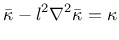(51)

with homogeneous Neumann boundary condition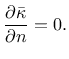(52)

In (51),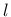is the length scale parameter and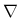is the Laplace operator.

The model description and parameters are summarized in Tabs. 11 and 12. Note that the internal length parameter r has the meaning of the radius of interactionfor the integral version (and thus has the dimension of length) but for the gradient version it has the meaning of the coefficient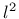multiplying the Laplacean in (51), and thus has the dimension of length squared.

Table 11: Nonlocal integral Mises plasticity - summary.
Description Nonlocal Mises plasticity with isotropic hardening
Record Format MisesMatNl (in) # d(rn) # E(rn) # n(rn) # sig0(rn) # H(rn) # omega_crit(rn) #a(rn) #r(rn) #m(rn) #[wft(in) #][scalingType(in) #]
Parameters - material number
- d material density
- E Young's modulus
- n Poisson's ratio
- sig0 initial yield stress in uniaxial tension (compression)
- H hardening modulus
- omega_crit critical damage
- a exponent in damage law
- r nonlocal interaction radiusfrom eq. (50)
- m over-nonlocal parameter
- wft selects the type of nonlocal weight function (see Section 1.5.7):
 1 - default, quartic spline (bell-shaped function with bounded support) 2 - Gaussian function 3 - exponential function (Green function in 1D) 4 - uniform averaging up to distance5 - uniform averaging over one finite element 6 - special function obtained by reducing the 2D exponential function to 1D (by numerical integration)
- scalingType selects the type of scaling of the weight function (e.g. near a boundary; see Section 1.5.7):
 1 - default, standard scaling with integral of weight function in the denominator 2 - no scaling (the weight function normalized in an infinite body is used even near a boundary) 3 - Borino scaling (local complement)
Supported modes 1dMat, PlaneStrain, 3dMat

Table 12: Gradient-enhanced Mises plasticity - summary.
 Description Gradient-enhanced Mises plasticity with isotropic damage Record Format MisesMatGrad (in) # d(rn) # E(rn) # n(rn) # sig0(rn) # H(rn) # omega_crit(rn) #a(rn) #r(rn) #m(rn) # Parameters - material number - d material density - E Young's modulus - n Poisson's ratio - sig0 initial yield stress in uniaxial tension (compression) - H hardening modulus - omega_crit critical damage - a exponent in damage law - r internal length scale parameterfrom eq. (51) - m over-nonlocal parameter Supported modes 1dMat, PlaneStrain, 3dMat

Borek Patzak
2019-03-19